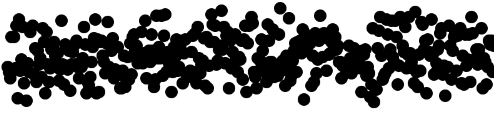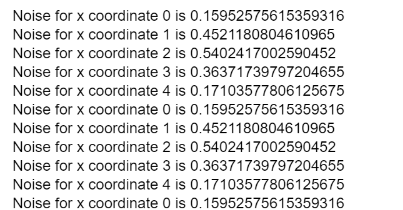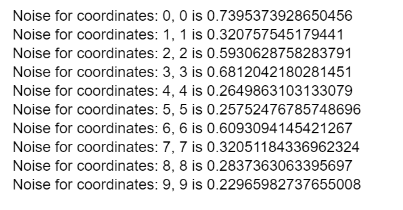# p5.js | noise() Function

• Last Updated : 29 Jan, 2020

The noise() function is used to return a number generated by Perlin noise at the given coordinates. This value is semi-random, which means that the value would be fixed for a coordinate for the lifespan of the program.

The Perlin noise value is different from the value returned by the random() function as this noise has a more natural and harmonic succession compared to the standard one.

Syntax:

`noise(x, [y], [z])`

Parameter: This function accept three parameters as mentioned above and described below:

• x: This is a number which represents the x-coordinate in the noise space.
• y: This is a number which represents the y-coordinate in the noise space. It is an optional parameter.
• z: This is a number which represents the z-coordinate in the noise space. It is an optional parameter.

Return Value: It returns the Perlin noise value between 0 and 1.

Below examples illustrates the noise() function in p5.js:

Example 1: Plotting the noise values of the y-coordinate of the moving point.

• Program:
 `let x_coordinate = 100.0;``let plot_x = 10.0;``  ` `function` `setup() {``    ``createCanvas(400, 200);``}``  ` `function` `draw() {``  ` `    ``// Get noise with x coordinate``    ``x_noise = noise(x_coordinate) * 100;``  ` `    ``// Plot the point with random noise``    ``strokeWeight(10);``    ``point(plot_x, x_noise);``  ` `    ``// Increment the x coordinate``    ``x_coordinate++;``  ` `    ``// Increase the x coordinate``    ``// for plotting``    ``plot_x++;``}`
• Output:Example 2: This example demonstrates the semi-random property of a function.

• Program:
 `let x_coordinate = 0.0;``let plot_y = 0.0;``  ` `function` `setup() {``    ``createCanvas(400, 200);``}``  ` `function` `draw() {``     ` `    ``if` `(x_coordinate < 5) {``  ` `        ``// Get noise with x coordinate``        ``x_noise = noise(x_coordinate);``    ` `        ``// Output the noise along with``        ``// its corresponding coordinate``        ``coord_text = ``"Noise for x coordinate "``            ``+ x_coordinate + ``" is "` `+ x_noise;``         ` `        ``text(coord_text, 10, plot_y);``  ` `        ``// Increment the x coordinate``        ``x_coordinate++;``  ` `        ``// Increase the y coordinate``        ``// for plotting``        ``plot_y = plot_y + 15;``    ``}``    ``else``        ``x_coordinate = 0;``}`
• Output:Example 3: This example uses two parameters for defining a point in the noise space.

• Program:
 `let x_coordinate = 0.0;``let y_coordinate = 0.0;``let plot_y = 0.0;``  ` `function` `setup() {``    ``createCanvas(400, 200);``}``  ` `function` `draw() {``     ` `    ``if` `(x_coordinate < 10) {``  ` `        ``// Get noise with x and y coordinates``        ``xy_noise = noise(x_coordinate, y_coordinate);``  ` `        ``// Output the noise along with``        ``// its corresponding coordinate``        ``coord_text = ``"Noise for coordinates: "``            ``+ x_coordinate + ``", "` `+ y_coordinate``            ``+ ``" is "` `+ xy_noise;``        ``text(coord_text, 10, plot_y);``  ` `        ``// Increment the x and y``        ``// coordinates``        ``x_coordinate++;``        ``y_coordinate++;``  ` `        ``// Increase the y coordinate``        ``// for plotting``        ``plot_y = plot_y + 15;``    ``}``}`
• Output:Online editor: https://editor.p5js.org/
Environment Setup: https://www.geeksforgeeks.org/p5-js-soundfile-object-installation-and-methods/

Reference: https://p5js.org/reference/#/p5/noise

My Personal Notes arrow_drop_up